# NCERT Exemplar solutions for Class 10 Mathematics chapter 12 - Surface Areas and Volumes [Latest edition]

#### Chapters## Solutions for Chapter 12: Surface Areas and Volumes

Below listed, you can find solutions for Chapter 12 of CBSE NCERT Exemplar for Class 10 Mathematics.

ExerciseExercise 12.1Exercise 12.2Exercise 12.3Exercise 12.4
Exercise

### NCERT Exemplar solutions for Class 10 Mathematics Chapter 12 Surface Areas and Volumes Exercise

Exercise | Q 1

What is the area of the largest triangle that can be fitted into a rectangle of length l units and width w units?

• (lw)/2

• (lw)/3

• (lw)/6

• (lw)/4

Exercise 12.1 [Pages 138 - 140]

### NCERT Exemplar solutions for Class 10 Mathematics Chapter 12 Surface Areas and Volumes Exercise 12.1 [Pages 138 - 140]

#### Choose the correct alternative:

Exercise 12.1 | Q 1 | Page 138

A cylindrical pencil sharpened at one edge is the combination of ______.

• a cone and a cylinder

• a hemisphere and a cylinder

• frustum of a cone and a cylinder

• two cylinders.

Exercise 12.1 | Q 2 | Page 138

A surahi is the combination of ______.

• a sphere and a cylinder

• a hemisphere and a cylinder

• two hemispheres

• a cylinder and a cone

Exercise 12.1 | Q 3 | Page 138

A plumbline (sahul) is the combination of (see figure) ______.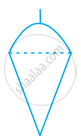• a cone and a cylinder

• a hemisphere and a cone

• frustum of a cone and a cylinder

• sphere and cylinder

Exercise 12.1 | Q 4 | Page 138

The shape of a glass (tumbler) (see figure) is usually in the form of ______.• a cone

• frustum of a cone

• a cylinder

• a sphere

Exercise 12.1 | Q 5 | Page 139

The shape of a gilli, in the gilli-danda game (see figure), is a combination of ______.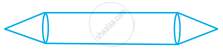• two cylinders

• a cone and a cylinder

• two cones and a cylinder

• two cylinders and a cone

Exercise 12.1 | Q 6 | Page 139

A shuttle cock used for playing badminton has the shape of the combination of ______.

• a cylinder and a sphere

• a cylinder and a hemisphere

• a sphere and a cone

• frustum of a cone and a hemisphere

Exercise 12.1 | Q 7 | Page 139

A cone is cut through a plane parallel to its base and then the cone that is formed on one side of that plane is removed. The new part that is leftover on the other side of the plane is called ______.

• A frustum of a cone

• Cone

• Cylinder

• Cylinder

Exercise 12.1 | Q 8 | Page 139

A hollow cube of internal edge 22 cm is filled with spherical marbles of diameter 0.5 cm and it is assumed that 1/8 space of the cube remains unfilled. Then the number of marbles that the cube can accomodate is ______.

• 142296

• 142396

• 142496

• 142596

Exercise 12.1 | Q 9 | Page 139

A metallic spherical shell of internal and external diameters 4 cm and 8 cm, respectively is melted and recast into the form a cone of base diameter 8cm. The height of the cone is ____________.

• 12 cm

• 14 cm

• 15 cm

• 18 cm

Exercise 12.1 | Q 10 | Page 139

A solid piece of iron in the form of a cuboid of dimensions 49 cm × 33 cm × 24 cm, is moulded to form a solid sphere. The radius of the sphere is ______

• 21 cm

• 23 cm

• 25 cm

• 19 cm

Exercise 12.1 | Q 11 | Page 139

A mason constructs a wall of dimensions 270 cm× 300 cm × 350 cm with the bricks each of size 22.5 cm × 11.25 cm × 8.75 cm and it is assumed that 1/8 space is covered by the mortar. Then the number of bricks used to construct the wall is ______.

• 11100

• 11200

• 11000

• 11300

Exercise 12.1 | Q 12 | Page 140

Twelve solid spheres of the same size are made by melting a solid metallic cylinder of base diameter 2 cm and height 16 cm. The diameter of each sphere is ______.

• 4 cm

• 3 cm

• 2 cm

• 6 cm

Exercise 12.1 | Q 13 | Page 140

The radii of the top and bottom of a bucket of slant height 45 cm are 28 cm and 7 cm, respectively. The curved surface area of the bucket is ______.

• 4950 cm2

• 4951 cm2

• 4952 cm2

• 4953 cm2

Exercise 12.1 | Q 14 | Page 140

A medicine-capsule is in the shape of a cylinder of diameter 0.5 cm with two hemispheres stuck to each of its ends. The length of entire capsule is 2 cm. The capacity of the capsule is ______.

• 0.36 cm3

• 0.35 cm3

• 0.34 cm3

• 0.33 cm3

Exercise 12.1 | Q 15 | Page 140

If two solid hemispheres of same base radius r are joined together along their bases, then curved surface area of this new solid is ______.

• 4πr2

• 6πr2

• 3πr2

• 8πr2

Exercise 12.1 | Q 16 | Page 140

A right circular cylinder of radius r cm and height h cm (h > 2r) just encloses a sphere of diameter ______.

• r cm

• 2r cm

• h cm

• 2h cm

Exercise 12.1 | Q 17 | Page 140

During conversion of a solid from one shape to another, the volume of the new shape will ______.

• Increase

• Decrease

• Remain unaltered

• Be doubled

Exercise 12.1 | Q 18 | Page 140

The diameters of the two circular ends of the bucket are 44 cm and 24 cm. The height of the bucket is 35 cm. The capacity of the bucket is ______.

• 32.7 litres

• 33.7 litres

• 34.7 litres

• 31.7 litres

Exercise 12.1 | Q 19 | Page 140

In a right circular cone, the cross-section made by a plane parallel to the base is a ______.

• Circle

• Frustum of a cone

• Sphere

• Hemisphere

Exercise 12.1 | Q 20 | Page 140

Volumes of two spheres are in the ratio 64:27. The ratio of their surface areas is ______.

• 3:4

• 4:3

• 9:16

• 16:9

Exercise 12.2 [Pages 142 - 143]

### NCERT Exemplar solutions for Class 10 Mathematics Chapter 12 Surface Areas and Volumes Exercise 12.2 [Pages 142 - 143]

#### State whether the following is True or False:

Exercise 12.2 | Q 1 | Page 142

Two identical solid hemispheres of equal base radius r cm are stuck together along their bases. The total surface area of the combination is 6πr2.

• True

• False

Exercise 12.2 | Q 2 | Page 142

A solid cylinder of radius r and height h is placed over other cylinder of same height and radius. The total surface area of the shape so formed is 4πrh + 4πr2.

• True

• False

Exercise 12.2 | Q 3 | Page 142

A solid cone of radius r and height h is placed over a solid cylinder having same base radius and height as that of a cone. The total surface area of the combined solid is pir [sqrt(r^2 + h^2) + 3r + 2h].

• True

• False

Exercise 12.2 | Q 4 | Page 142

A solid ball is exactly fitted inside the cubical box of side a. The volume of the ball is 4/3 pia^3.

• True

• False

Exercise 12.2 | Q 5 | Page 142

The volume of the frustum of a cone is 1/3 pi/h [r_1^2 + r_2^2 - r_1r_2], where h is vertical height of the frustum and r1, r2 are the radii of the ends.

• True

• False

Exercise 12.2 | Q 6 | Page 142

The capacity of a cylindrical vessel with a hemispherical portion raised upward at the bottom as shown in the figure is (pir^2)/3 [3h - 2r].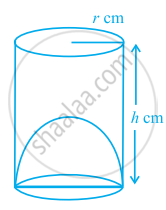• True

• False

Exercise 12.2 | Q 7 | Page 143

The curved surface area of a frustum of a cone is πl (r1 + r2), where l = sqrt(h^2 + (r_1 + r_2)^2), r1 and r2 are the radii of the two ends of the frustum and h is the vertical height.

• True

• False

Exercise 12.2 | Q 8 | Page 143

An open metallic bucket is in the shape of a frustum of a cone, mounted on a hollow cylindrical base made of the same metallic sheet. The surface area of the metallic sheet used is equal to curved surface area of frustum of a cone + area of circular base + curved surface area of cylinder.

• True

• False

Exercise 12.3 [Pages 146 - 147]

### NCERT Exemplar solutions for Class 10 Mathematics Chapter 12 Surface Areas and Volumes Exercise 12.3 [Pages 146 - 147]

Exercise 12.3 | Q 1 | Page 146

Three metallic solid cubes whose edges are 3 cm, 4 cm and 5 cm are melted and formed into a single cube. Find the edge of the cube so formed.

Exercise 12.3 | Q 2 | Page 146

How many shots each having diameter 3 cm can be made from a cuboidal lead solid of dimensions 9 cm × 11cm × 12cm?

Exercise 12.3 | Q 3 | Page 146

A bucket is in the form of a frustum of a cone and holds 28.490 litres of water. The radii of the top and bottom are 28 cm and 21 cm, respectively. Find the height of the bucket.

Exercise 12.3 | Q 4 | Page 146

A cone of radius 8 cm and height 12 cm is divided into two parts by a plane through the mid-point of its axis parallel to its base. Find the ratio of the volumes of two parts.

Exercise 12.3 | Q 5 | Page 146

Two identical cubes each of volume 64 cm3 are joined together end to end. What is the surface area of the resulting cuboid?

Exercise 12.3 | Q 6 | Page 146

From a solid cube of side 7 cm, a conical cavity of height 7 cm and radius 3 cm is hollowed out. Find the volume of the remaining solid.

Exercise 12.3 | Q 7 | Page 146

Two cones with same base radius 8 cm and height 15 cm are joined together along their bases. Find the surface area of the shape so formed.

Exercise 12.3 | Q 8 | Page 146

Two solid cones A and B are placed in a cylinderical tube as shown in the figure. The ratio of their capacities are 2:1. Find the heights and capacities of cones. Also, find the volume of the remaining portion of the cylinder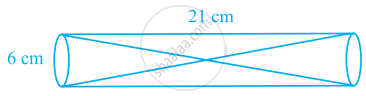Exercise 12.3 | Q 9 | Page 146

An ice cream cone full of ice cream having radius 5 cm and height 10 cm as shown in the figure. Calculate the volume of ice cream, provided that its 1/6 part is left unfilled with ice cream.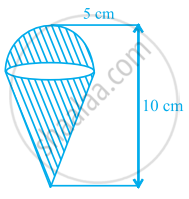Exercise 12.3 | Q 10 | Page 147

Marbles of diameter 1.4 cm are dropped into a cylindrical beaker of diameter 7 cm containing some water. Find the number of marbles that should be dropped into the beaker so that the water level rises by 5.6 cm.

Exercise 12.3 | Q 11 | Page 147

How many spherical lead shots each of diameter 4.2 cm can be obtained from a solid rectangular lead piece with dimensions 66 cm, 42 cm and 21 cm.

Exercise 12.3 | Q 12 | Page 147

How many spherical lead shots of diameter 4 cm can be made out of a solid cube of lead whose edge measures 44 cm.

Exercise 12.3 | Q 13 | Page 147

A wall 24 m long, 0.4 m thick and 6 m high is constructed with the bricks each of dimensions 25 cm × 16 cm × 10 cm. If the mortar occupies 1/10 th of the volume of the wall, then find the number of bricks used in constructing the wall.

Exercise 12.3 | Q 14 | Page 147

Find the number of metallic circular disc with 1.5 cm base diameter and of height 0.2 cm to be melted to form a right circular cylinder of height 10 cm and diameter 4.5 cm.

Exercise 12.4 [Pages 150 - 152]

### NCERT Exemplar solutions for Class 10 Mathematics Chapter 12 Surface Areas and Volumes Exercise 12.4 [Pages 150 - 152]

Exercise 12.4 | Q 1 | Page 150

A solid metallic hemisphere of radius 8 cm is melted and recasted into a right circular cone of base radius 6 cm. Determine the height of the cone.

Exercise 12.4 | Q 2 | Page 150

A rectangular water tank of base 11 m × 6 m contains water upto a height of 5 m. If the water in the tank is transferred to a cylindrical tank of radius 3.5 m, find the height of the water level in the tank.

Exercise 12.4 | Q 3 | Page 150

How many cubic centimetres of iron is required to construct an open box whose external dimensions are 36 cm, 25 cm and 16.5 cm provided the thickness of the iron is 1.5 cm. If one cubic cm of iron weighs 7.5 g, find the weight of the box.

Exercise 12.4 | Q 4 | Page 150

The barrel of a fountain pen, cylindrical in shape, is 7 cm long and 5 mm in diameter. A full barrel of ink in the pen is used up on writing 3300 words on an average. How many words can be written in a bottle of ink containing one fifth of a litre?

Exercise 12.4 | Q 5 | Page 150

Water flows at the rate of 10m/minute through a cylindrical pipe 5 mm in diameter. How long would it take to fill a conical vessel whose diameter at the base is 40 cm and depth 24 cm?

Exercise 12.4 | Q 6 | Page 150

A heap of rice is in the form of a cone of diameter 9 m and height 3.5 m. Find the volume of the rice. How much canvas cloth is required to just cover the heap?

Exercise 12.4 | Q 7 | Page 150

A factory manufactures 120000 pencils daily. The pencils are cylindrical in shape each of length 25 cm and circumference of base as 1.5 cm. Determine the cost of colouring the curved surfaces of the pencils manufactured in one day at Rs 0.05 per dm2.

Exercise 12.4 | Q 8 | Page 151

Water is flowing at the rate of 15 km/hour through a pipe of diameter 14 cm into a cuboidal pond which is 50 m long and 44 m wide. In what time will the level of water in the pond rise by 21 cm?

Exercise 12.4 | Q 9 | Page 151

A solid iron cuboidal block of dimensions 4.4 m × 2.6 m × 1m is recast into a hollow cylindrical pipe of internal radius 30 cm and thickness 5 cm. Find the length of the pipe.

Exercise 12.4 | Q 10 | Page 151

500 persons are taking a dip into a cuboidal pond which is 80 m long and 50 m broad. What is the rise of water level in the pond, if the average displacement of the water by a person is 0.04m3?

Exercise 12.4 | Q 11 | Page 151

16 glass spheres each of radius 2 cm are packed into a cuboidal box of internal dimensions 16 cm × 8 cm × 8 cm and then the box is filled with water. Find the volume of water filled in the box.

Exercise 12.4 | Q 12 | Page 151

A milk container of height 16 cm is made of metal sheet in the form of a frustum of a cone with radii of its lower and upper ends as 8 cm and 20 cm respectively. Find the cost of milk at the rate of Rs. 22 per litre which the container can hold.

Exercise 12.4 | Q 13 | Page 151

A cylindrical bucket of height 32 cm and base radius 18 cm is filled with sand. This bucket is emptied on the ground and a conical heap of sand is formed. If the height of the conical heap is 24 cm, find the radius and slant height of the heap.

Exercise 12.4 | Q 14 | Page 151

A rocket is in the form of a right circular cylinder closed at the lower end and surmounted by a cone with the same radius as that of the cylinder. The diameter and height of the cylinder are 6 cm and 12 cm, respectively. If the slant height of the conical portion is 5 cm, find the total surface area and volume of the rocket [Use π = 3.14].

Exercise 12.4 | Q 15 | Page 151

A building is in the form of a cylinder surmounted by a hemispherical vaulted dome and contains 41 19/21 m^3 of air. If the internal diameter of dome is equal to its total height above the floor, find the height of the building?

Exercise 12.4 | Q 16 | Page 151

A hemispherical bowl of internal radius 9 cm is full of liquid. The liquid is to be filled into cylindrical shaped bottles each of radius 1.5 cm and height 4 cm. How many bottles are needed to empty the bowl?

Exercise 12.4 | Q 17 | Page 151

A solid right circular cone of height 120 cm and radius 60 cm is placed in a right circular cylinder full of water of height 180 cm such that it touches the bottom. Find the volume of water left in the cylinder, if the radius of the cylinder is equal to the radius of the cone.

Exercise 12.4 | Q 18 | Page 152

Water flows through a cylindrical pipe, whose inner radius is 1 cm, at the rate of 80 cm/sec in an empty cylindrical tank, the radius of whose base is 40 cm. What is the rise of water level in tank in half an hour?

Exercise 12.4 | Q 19 | Page 152

The rain water from a roof of dimensions 22 m × 20 m drains into a cylindrical vessel having diameter of base 2 m and height 3.5 m. If the rain water collected from the roof just fill the cylindrical vessel, then find the rainfall in cm.

Exercise 12.4 | Q 20 | Page 152

A pen stand made of wood is in the shape of a cuboid with four conical depressions and a cubical depression to hold the pens and pins, respectively. The dimension of the cuboid are 10 cm, 5 cm and 4 cm. The radius of each of the conical depressions is 0.5 cm and the depth is 2.1 cm. The edge of the cubical depression is 3 cm. Find the volume of the wood in the entire stand.

## Solutions for Chapter 12: Surface Areas and Volumes

ExerciseExercise 12.1Exercise 12.2Exercise 12.3Exercise 12.4## NCERT Exemplar solutions for Class 10 Mathematics chapter 12 - Surface Areas and Volumes

Shaalaa.com has the CBSE Mathematics Class 10 Mathematics CBSE solutions in a manner that help students grasp basic concepts better and faster. The detailed, step-by-step solutions will help you understand the concepts better and clarify any confusion. NCERT Exemplar solutions for Mathematics Class 10 Mathematics CBSE 12 (Surface Areas and Volumes) include all questions with answers and detailed explanations. This will clear students' doubts about questions and improve their application skills while preparing for board exams.

Further, we at Shaalaa.com provide such solutions so students can prepare for written exams. NCERT Exemplar textbook solutions can be a core help for self-study and provide excellent self-help guidance for students.

Concepts covered in Class 10 Mathematics chapter 12 Surface Areas and Volumes are Concept of Surface Area, Volume, and Capacity, Surface Area of a Combination of Solids, Surface Area and Volume of Different Combination of Solid Figures, Surface Area and Volume of Three Dimensional Figures, Volume of a Combination of Solids, Conversion of Solid from One Shape to Another, Frustum of a Cone, Concept of Surface Area, Volume, and Capacity.

Using NCERT Exemplar Class 10 Mathematics solutions Surface Areas and Volumes exercise by students is an easy way to prepare for the exams, as they involve solutions arranged chapter-wise and also page-wise. The questions involved in NCERT Exemplar Solutions are essential questions that can be asked in the final exam. Maximum CBSE Class 10 Mathematics students prefer NCERT Exemplar Textbook Solutions to score more in exams.

Get the free view of Chapter 12, Surface Areas and Volumes Class 10 Mathematics additional questions for Mathematics Class 10 Mathematics CBSE, and you can use Shaalaa.com to keep it handy for your exam preparation.

Share